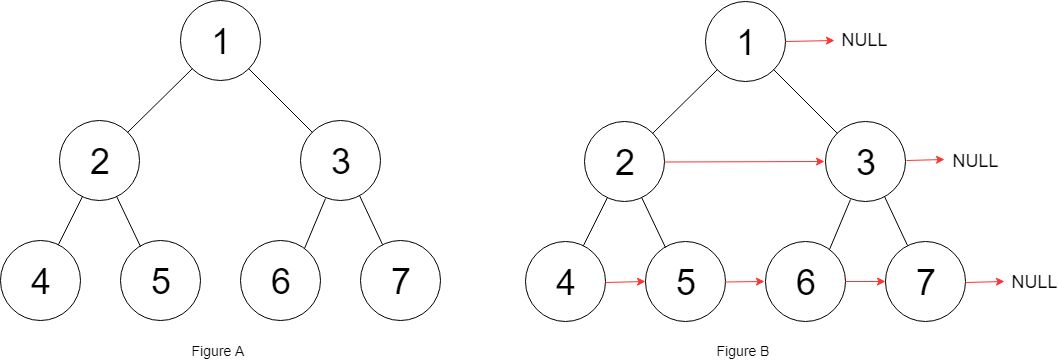0116. Populating Next Right Pointers in Each Node

# 116. Populating Next Right Pointers in Each Node#

## 题目 #

You are given a perfect binary tree where all leaves are on the same level, and every parent has two children. The binary tree has the following definition:

``````struct Node {
int val;
Node *left;
Node *right;
Node *next;
}

``````

Populate each next pointer to point to its next right node. If there is no next right node, the next pointer should be set to `NULL`.

Initially, all next pointers are set to `NULL`.

• You may only use constant extra space.
• Recursive approach is fine, you may assume implicit stack space does not count as extra space for this problem.

Example 1:``````Input: root = [1,2,3,4,5,6,7]
Output: [1,#,2,3,#,4,5,6,7,#]
Explanation:Given the above perfect binary tree (Figure A), your function should populate each next pointer to point to its next right node, just like in Figure B. The serialized output is in level order as connected by the next pointers, with '#' signifying the end of each level.

``````

Constraints:

• The number of nodes in the given tree is less than `4096`.
• `1000 <= node.val <= 1000`

## 题目大意 #

``````struct Node {
int val;
Node *left;
Node *right;
Node *next;
}

``````

## 解题思路 #

• 本质上是二叉树的层序遍历，基于广度优先搜索，将每层的节点放入队列，并遍历队列进行连接。

## 代码 #

``````package leetcode

type Node struct {
Val   int
Left  *Node
Right *Node
Next  *Node
}

//解法一：迭代
func connect(root *Node) *Node {
if root == nil {
return root
}
q := []*Node{root}
for len(q) > 0 {
var p []*Node
// 遍历这一层的所有节点
for i, node := range q {
if i+1 < len(q) {
node.Next = q[i+1]
}
if node.Left != nil {
p = append(p, node.Left)
}
if node.Right != nil {
p = append(p, node.Right)
}
}
q = p
}
return root
}

// 解法二 递归
func connect2(root *Node) *Node {
if root == nil {
return nil
}
connectTwoNode(root.Left, root.Right)
return root
}

func connectTwoNode(node1, node2 *Node) {
if node1 == nil || node2 == nil {
return
}
node1.Next = node2
connectTwoNode(node1.Left, node1.Right)
connectTwoNode(node2.Left, node2.Right)
connectTwoNode(node1.Right, node2.Left)
}
``````Apr 8, 2023Edit this page